# Raspberry Pi Based Oscilloscope

Published  April 11, 2018   12
E
Author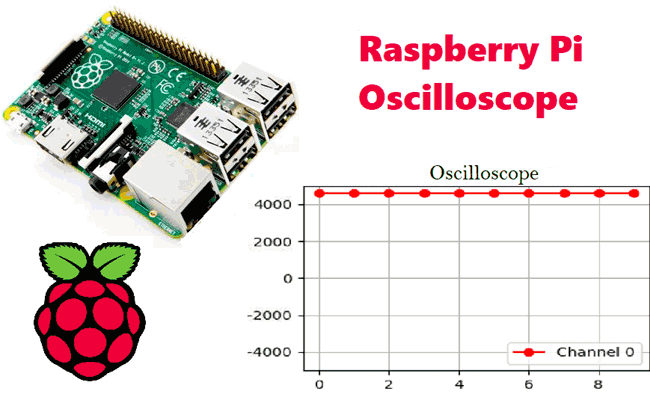Hi guys, welcome to today’s post. One of the most fascinating thing about being a maker is knowing how to develop makeshift tools, you will never get stuck working on any project when you have that kind of versatility. So Today, I will be sharing how to build a Raspberry Pi based makeshift version of one of the most important tools in Electrical/Electronics engineering; The Oscilloscope.

The oscilloscope is an electronic test instrument that allows the visualization and observation of varying signal voltages, usually as a two dimensional plot with one or more signals plotted against time. Today’s project will seek to replicate the signal visualization capabilities of the oscilloscope using the Raspberry Pi and an analog to digital converter module.

### Project Flow:

Replicating the signal visualization of the oscilloscope using the Raspberry Pi will require the following steps;

1. Perform Digital to analog conversion of the Input signal

2. Prepare the resulting data for representation

3. Plot the data on a live time graph

A simplified block diagram for this project would look like the diagram below.### Project Requirements

The requirement for this project can be classified into two:

1. Hardware Requirements
2. Software Requirements

Hardware requirements

To build this project, the following components/part are required;

1. Raspberry pi 2 (or any other model)
2. 8 or 16GB SD Card
3. LAN/Ethernet Cable
4. Power Supply or USB cable
6. LDR (Optional as its meant for test)
7. 10k or 1k resistor
8. Jumper wires
10. Monitor or any other way of seeing the pi’s Desktop(VNC inclusive)

Software Requirements

The software requirements for this project are basically the python modules (matplotlib and drawnow) that will be used for data visualization and the Adafruit module for interfacing with the ADS1115 ADC chip. I will show how to install these modules on the Raspberry Pi as we proceed.

While this tutorial will work irrespective of the raspberry pi OS used, I will be using the Raspberry Pi stretch OS and I will assume you are familiar with setting up the Raspberry Pi with the Raspbian stretch OS, and you know how to SSH into the raspberry pi using a terminal software like putty. If you have issues with any of this, there are tons of Raspberry Pi Tutorials on this website that can help.

With all the hardware components in place, let's create the schematics and connect the components together.

### Circuit Diagram:

The ADC is an I2C based device and should be connected to the Raspberry Pi as shown in the schematics below.

For clarity, the pin connection between the two components is also described below.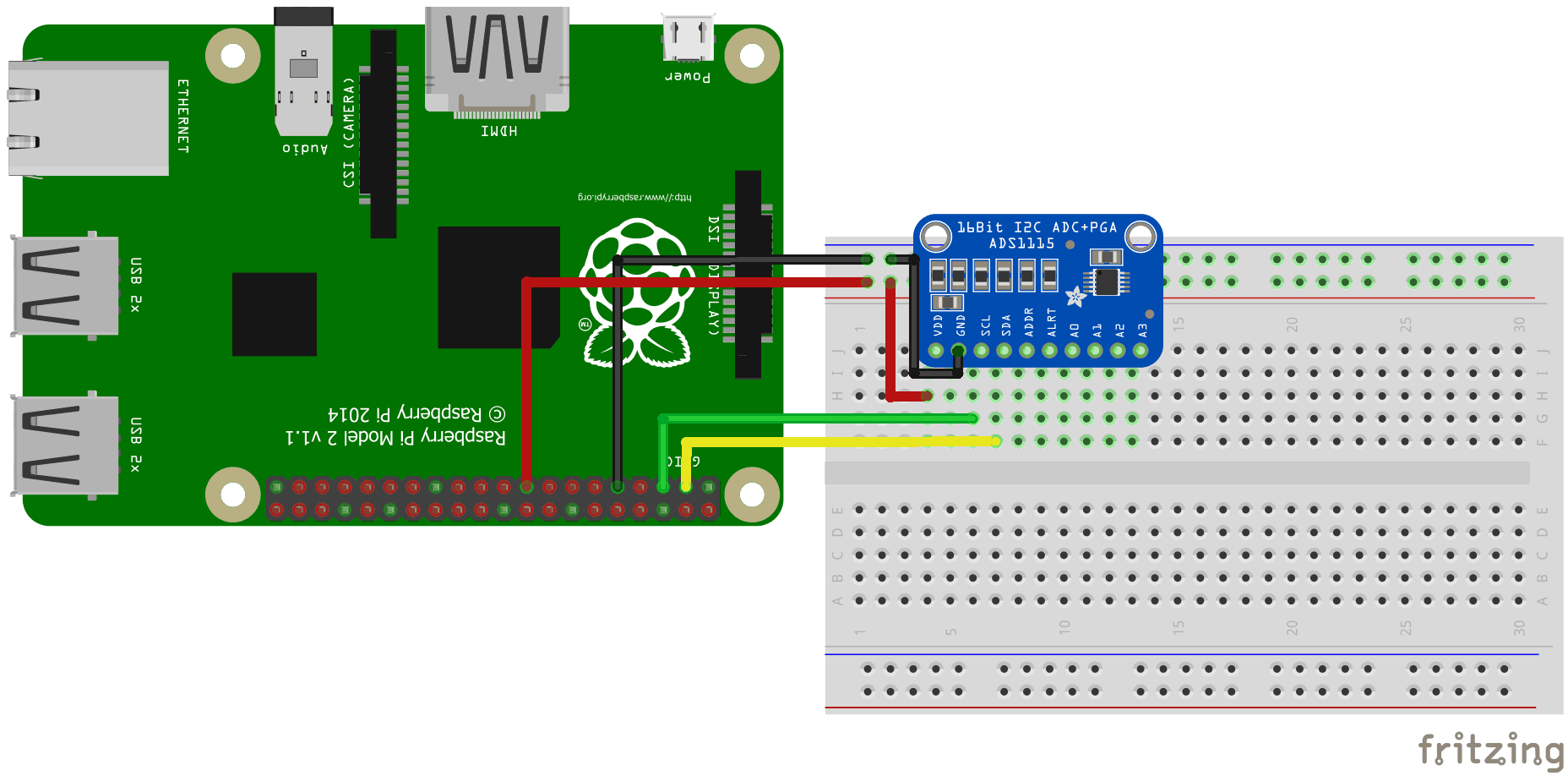VDD – 3.3v

GND – GND

SDA – SDA

SCL – SCL

With the connections all done, power up your pi and proceed to install the dependencies mentioned below.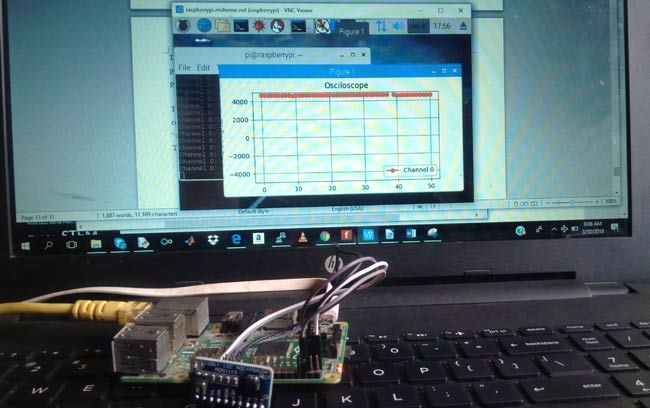### Install Dependencies for Raspberry Pi Oscilloscope:

Before we start writing the python script to pull data from the ADC and plot it on a live graph, we need to enable the I2C communication interface of the raspberry pi and install the software requirements that were mentioned earlier. This will be done in below steps so its easy to follow:

Step 1: Enable Raspberry Pi I2C interface

To enable the I2C, from the terminal, run;

`sudo raspi-config`

When the configuration panels open, select interface options, select I2C and click enable.

Step 2: Update the Raspberry pi

The first thing I do before starting any project is updating the Pi. Through this, I am sure every thing on the OS is up to date and I won’t experience compatibility issue with any latest software I choose to install on the Pi. To do this, run below two commands:

```sudo apt-get update

With the update done, we are now ready to install the dependencies starting with the Adafruit python module for the ADS115 chip. Ensure you are in the Raspberry Pi home directory by running;

`cd ~`

then install the build-essentials by running;

`sudo apt-get install build-essential python-dev python-smbus git`

Next, clone the Adafruit git folder for the library by running;

`git clone https://github.com/adafruit/Adafruit_Python_ADS1x15.git`

Change into the cloned file’s directory and run the setup file;

```cd Adafruit_Python_ADS1x1z
sudo python setup.py install
```

After installation, your screen should look like the image below.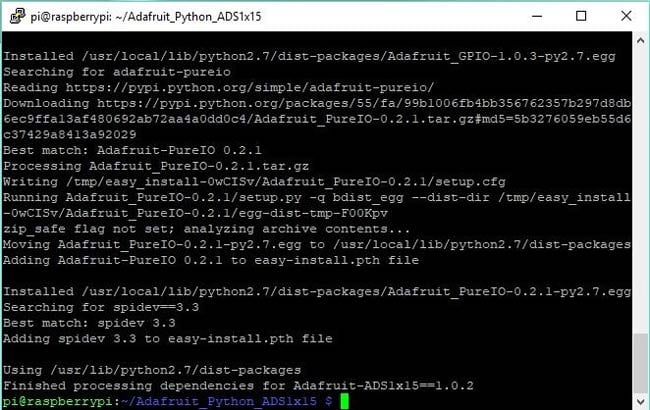Step 4: Test the library and 12C communication.

Before we proceed with the rest of the project, it is important to test the library and ensure the ADC can communicate with the raspberry pi over I2C. To do this we will use an example script that comes with the library.

While still in the Adafruit_Python_ADS1x15 folder, change directory to the examples directory by running;

`cd examples`

Next, run the sampletest.py example which displays the value of the four channels on the ADC in a tabular form.

Run the example using:

`python simpletest.py`

If the I2C module is enabled and connections good, you should see the data as shown in the image below.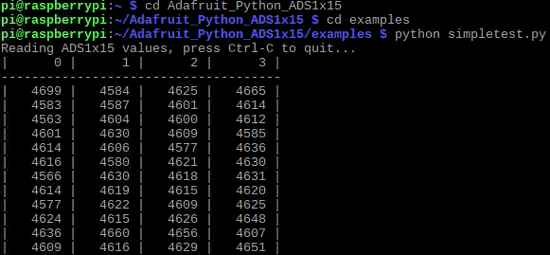If an error occurs, check to ensure the ADC is well connected to the PI and I2C communication is enabled on the Pi.

Step 5: Install Matplotlib

To visualize the data we need to install the matplotlib module which is used to plot all kind of graphs in python. This can be done by running;

`sudo apt-get install python-matplotlib`

You should see an outcome like the image below.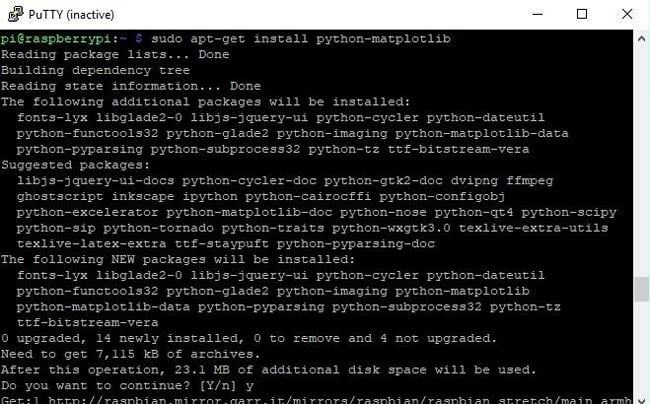Step6: Install the Drawnow python module

Lastly, we need to install the drawnow python module. This module helps us provide live updates to the data plot.

We will be installing drawnow via the python package installer; pip, so we need to ensure it is installed.  This can be done by running;

`sudo apt-get install python-pip`

We can then use pip to install the drawnow package by running:

`sudo pip install drawnow`

You should get an outcome like the image below after running it.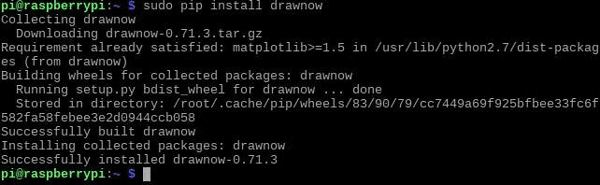With all the dependencies installed, we are now ready to write the code.

### Python Code for Raspberry Pi Oscilloscope:

The python code for this Pi Oscilloscope is fairly simple especially if you are familiar with the python matplotlib module. Before showing us the whole code, I will try to break it into part and explain what each part of the code is doing so you can have enough knowledge to extend the code to do more stuffs.

At this stage it is important to switch to a monitor or use the VNC viewer, anything through which you can see your Raspberry Pi’s desktop, as the graph being plotted won’t show on the terminal.

With the monitor as the interface open a new python file. You can call it any name you want, but I will call it scope.py.

`sudo nano scope.py`

With the file created, the first thing we do is import the modules we will be using;

```import time
import matplotlib.pyplot as plt
from drawnow import *
```

```adc = Adafruit_ADS1x15.ADS1115()
```

Next, we set the gain of the ADC. There are different ranges of gain and should be chosen based on the voltage you are expecting at the input of the ADC. For this tutorial, we are estimating a 0 – 4.09v so we will be using a gain of 1. For more info on gain you can check the ADS1015/ADS1115 datasheet.

`GAIN = 1`

Next, we need to create the array variables that will be used to store the data to be plotted and another one to serve as count.

```Val = [ ]
cnt = 0
```

Next, we make know our intentions of making the plot interactive known so as to enable us plot the data live.

```plt.ion()
```

Next, we start continuous ADC conversion specifying the ADC channel, in this case, channel 0 and we also specify the gain.

It should be noted that all the four ADC channels on the ADS1115 can be read at the same time, but 1 channel is enough for this demonstration.

```adc.start_adc(0, gain=GAIN)
```

Next we create a function def makeFig, to create and set the attributes of the graph which will hold our live plot. We first of all set the limits of the y-axis using ylim, after which we input the title of the plot, and the label name before we specify the data that will be plotted and its plot style and color using plt.plot(). We can also state the channel (as channel 0 was stated) so we can identify each signal when the four channels of the ADC are being used.  plt.legend is used to specify where we want the information about that signal(e.g Channel 0) displayed on the figure.

``` plt.ylim(-5000,5000)
plt.title('Osciloscope')
plt.grid(True)
plt.plot(val, 'ro-', label='lux')
plt.legend(loc='lower right')
```

Next we write the while loop which will be used constantly read data from the ADC and update the plot accordingly.

```value = adc.get_last_result()
```

Next we print the value on the terminal just to give us another way of confirming the plotted data. We wait a few seconds after printing then we append the data to the list (val) created to store the data for that channel.

```print('Channel 0: {0}'.format(value))
time.sleep(0.5)
val.append(int(value))```

We then call drawnow to update the plot.

`drawnow(makeFig)`

To ensure the latest data is what is available on the plot, we delete the data at index 0 after every 50 data counts.

```cnt = cnt+1
if(cnt>50):
val.pop(0)```

That’s all!

The complete Python code is given at the end of this tutorial.

### Raspberry Pi Oscilloscope in Action:

Copy the complete python code and paste in the python file we created earlier, remember we will need a monitor to view the plot so all of this should be done by either VNC or with a connected monitor or screen.

Save the code and run using;

```sudo python scope.py
```

If you used a different name other than scope.py, don’t forget to change this to match.

After a few minutes, you should see the ADC data being printed on the terminal. Occasionally you may get a warning from matplotlib (as shown in the image below) which should be suppressed but it doesn’t affect the data being displayed or the plot in anyway. To suppress the warning however, the following lines of code can be added after the import lines in our code.

```Import warnings
import matplotlib.cbook
warnings.filterwarnings(“ignore”, category=matplotlib.cbook.mplDeprecation)```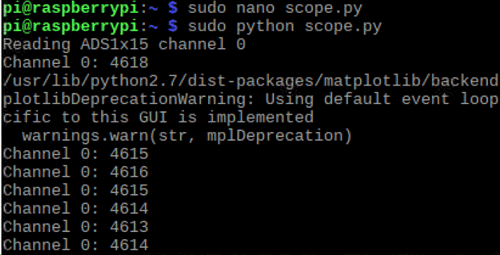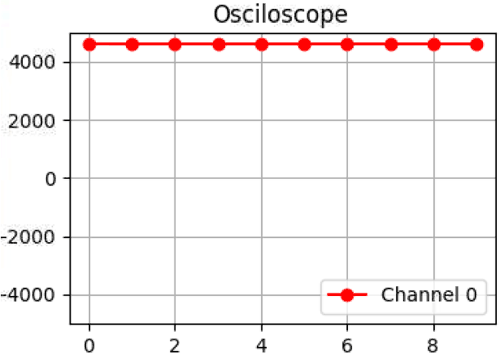That’s it for this tutorial guys, to fully test your oscilloscope, you can connect an analog device like a potentiometer to a channel on the ADC and you should see the data change with each turn of the potentiometer. Or you can input Sine wave or square wave to test the output.

Thanks for reading, if you have any question(s) or something you will like me to add, just leave me a comment.

Till next time, Keep making!

Code

import time
import matplotlib.pyplot as plt
#import numpy
from drawnow import *

GAIN = 1
val = [ ]
cnt = 0
plt.ion()
# Start continuous ADC conversions on channel 0 using the previous gain value.
#create the figure function
def makeFig():
plt.ylim(-5000,5000)
plt.title('Osciloscope')
plt.grid(True)
plt.plot(val, 'ro-', label='Channel 0')
plt.legend(loc='lower right')
while (True):
print('Channel 0: {0}'.format(value))
# Sleep for half a second.
time.sleep(0.5)
val.append(int(value))
drawnow(makeFig)
plt.pause(.000001)
cnt = cnt+1
if(cnt>50):
val.pop(0)

Tags

Submitted by Timothy Wolfram on Wed, 04/11/2018 - 21:58

### Wow nice project.

I love your project, I am going to have to build this in the very near future. Thanks!!!

Submitted by Aje Joseph on Mon, 04/16/2018 - 01:06

### Thanks for this.

Thanks so much for this bro, you never cease to inspire me. Well done

Submitted by Anudeep n Rao on Tue, 04/17/2018 - 19:14

### What is the baud rate you are

What is the baud rate you are operating it at and what is the maximum frequency you have been able to observe properly?

Submitted by Dakota on Mon, 05/21/2018 - 00:32

### Time change

How do I make the scope work in real-time. It seems to lag a good bit. I am using the pi zero w. Thanks

Submitted by Shubham Nath on Wed, 07/04/2018 - 16:26

### I want to measure voltage of

I want to measure voltage of a simple battery (cell). After researching and googling, measuring the battery voltage require an adc. I want to send the voltage data to cloud. A0 and A1 pins are used to find the potential difference (voltage) of one battery.
Will this work?

Submitted by manorama darekar on Tue, 08/07/2018 - 18:25

### query ...plz help

nice tutorial sir ... i follow each step and my script is executing successfully ... but unable to display graph on screen ... I already installed matplotlib. plz help me as I am new to all this .. currently using raspberry pi 3 , Python 3.5

### Because pi has two pythons usually

The two pythons are python 2 and python 3

if you want matplotlib to work with python 3. When downloading pip has to be pip3 and you need to specify python3 rather than python.

So the above instructions are

So for python3

sudo apt-get install build-essential python-dev python-smbus git
sudo python setup.py install
python simpletest.py
sudo apt-get install python-matplotlib
sudo apt-get install python-pip
sudo pip install drawnow

becomes

sudo apt-get install build-essential python3-dev python3-smbus git
sudo python3 setup.py install
python3 simpletest.py
sudo apt-get install python3-matplotlib
sudo apt-get install python3-pip
sudo pip3 install drawnow

I have a MCP3008 chip so have altered your program to suit.

#!/usr/bin/python

import spidev
import time
import os
import matplotlib.pyplot as plt

from drawnow import *
#GAIN = 1
val = [ ]
val1 = [ ]
cnt = 0
plt.ion()

# Open SPI bus
spi = spidev.SpiDev()
spi.open(0,0)
spi.max_speed_hz=1000000

# Function to read SPI data from MCP3008 chip
# Channel must be an integer 0-7
return data

# Function to convert data to voltage level,
# rounded to specified number of decimal places.
def ConvertVolts(data,places):
volts = (data * 3.3) / float(1023)
volts = round(volts,places)
return volts

def makeFig1():
plt.ylim(-30,800)
plt.xlim(0,101)
plt.title('Osciloscope1')
plt.grid(True)
plt.ylabel('Light Level')
plt.plot(val, 'ro-', label='Light Level')

plt.legend(loc='upper left')

def makeFig2():
plt.ylim(-1,3)
plt.xlim(0,101)
plt.title('Osciloscope2')
plt.grid(True)
plt.ylabel('Voltage')

plt.plot(val1, 'go-', label='Voltage Level')
plt.legend(loc='upper left')

# Define sensor channels
light_channel = 0

delay = 0.01
drawnow(makeFig1)
while True:

# Read the light sensor data
light_volts = ConvertVolts(light_level,2)
value = light_level
value1 = light_volts

# Sleep for half a second.

time.sleep(delay)
val.append(int(value))
val1.append(int(value1))
drawnow(makeFig1)
drawnow(makeFig2)
plt.pause(.000001)
cnt = cnt+1
if(cnt>100):
val.pop(0)
val1.pop(0)

# Print out results

print("Light: {} ({}V)".format(light_level,light_volts))

### Good Work! But it's been too

Good Work! But it's been too slow to show higher frequencies than 0,5Hz.

I've changed the code a little bit, because the "drawnow" function takes too much time.

import time
import matplotlib.pyplot as plt
#import numpy
from drawnow import *

GAIN = 1
val = [ ]
plt.ion()
# Start continuous ADC conversions on channel 0 using the previous gain value.
#create the figure function
def makeFig():
plt.ylim(-5000,5000)
plt.title('Oscilloscope')
plt.grid(True)
plt.plot(val, 'ro-', label='Channel 0')
plt.legend(loc='lower right')
while (True):
for i in range(200):